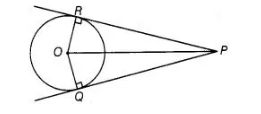# Prove that the centre of a circle

Question:

Prove that the centre of a circle touching two intersecting lines lies on the angle bisector of the lines.

Solution:

Given Two tangents PQ and PR are drawn from an external point P to a circle with centre 0.To prove Centre of a circle touching two intersecting lines lies on the angle bisector of the lines.
In ∠RPQ.

Construction Join $\mathrm{OR}$, and $\mathrm{OQ}$.

In $\triangle \mathrm{POP}$ and $\triangle \mathrm{POO}$

$\angle P R O=\angle P Q O=90^{\circ}$

[tangent at any point of a circle is perpendicular to the radius through the point of contact]

$O R=O Q$ [radii of some circle]

Since OP is common

$\therefore \quad \triangle P R O \cong \triangle P Q O \quad$ [RHS]

Hence, $\angle R P O=\angle Q P O$ [by CPCT]

Thus, $O$ lies on angle bisecter of $P R$ and $P Q$.

Hence proved.